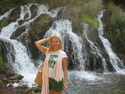The best dating service in Russia and Ukraine

## Become a Member

You can register and become a member. It's completely FREE.

Register

## Search Profiles

 I am a: female male seeking a: female male with photos only age from: 18192021222324252627282930313233343536373839404142434445464748495051525354555657585960616263646566676869707172737475  to  18192021222324252627282930313233343536373839404142434445464748495051525354555657585960616263646566676869707172737475 country: All countriesAmerican SamoaAndorraAnguillaAntarcticaAntigua and BarbudaArgentinaArmeniaArubaAustraliaAustriaAzerbaijanBahamasBahrainBarbadosBelarusBelgiumBelizeBeninBermudaBhutanBoliviaBosnia/HerzegowinaBotswanaBouvet IslandBrazilBritish Ind. OceanBrunei DarussalamBulgariaBurkina FasoCameroonCanadaCape VerdeCayman IslandsChadChileChinaChristmas IslandCocoa (Keeling) Is.ColombiaComorosCosta RicaCote DivoireCroatiaCubaCyprusCzech RepublicDenmarkDjiboutiDominicaDominican RepublicEcuadorEgyptEl SalvadorEquatorial GuineaEstoniaFalkland IslandsFaroe IslandsFijiFinlandFranceGabonGambiaGeorgiaGermanyGhanaGibraltarGreeceGreenlandGrenadaGuadeloupeGuamGuatemalaGuineaGuinea-BissauGuyanaHondurasHong KongHungaryIcelandIndiaIndonesiaIranIrelandIsraelItalyJamaicaJapanJordanKazakhstanKenyaKiribatiKoreaKuwaitKyrgyzstanLatviaLebanonLesothoLiechtensteinLithuaniaLuxembourgMacauMacedoniaMadagascarMalawiMalaysiaMaldivesMaliMaltaMarshall IslandsMartiniqueMauritaniaMauritiusMayotteMexicoMicronesiaMoldovaMonacoMontenegroMontserratMoroccoMozambiqueNamibiaNepalNetherlandsNew CaledoniaNew ZealandNicaraguaNigerNiueNorfolk IslandNorwayOmanPakistanPalauPanamaPapua New GuineaParaguayPeruPhilippinesPitcairnPolandPortugalPuerto RicoQatarReunionRomaniaRussiaSaint LuciaSamoaSan MarinoSaudi ArabiaSenegalSerbiaSeychellesSingaporeSlovakiaSloveniaSolomon IslandsSomaliaSouth AfricaSpainSri LankaSt. HelenaSwazilandSwedenSwitzerlandTaiwanTajikistanTanzaniaThailandTogoTokelauTongaTrinidad and TobagoTunisiaTurkeyTurkmenistanTuvaluUSAUgandaUkraineUnited Arab EmiratesUnited KingdomUruguayUzbekistanVanuatuVaticanVenezuelaViet NamVirgin IslandsWestern SaharaYemenZambia
 or search by profile ID:

# poustovit - 54 y/o female, Zodiac: Pisces from kiev, Ukrainemore photos
poustovit
• 54 y/o female, Zodiac: Pisces
• kiev, Ukraine
• English(Intermediate), Russian(Fluent)
• 1 child
• Last online: 2017-12-24 18:48:14
• ID: 1000240952

 Private details and contact information Personal details Sex female Children 1 child Want children I will tell you later Height 5'4" - 5'5" (161-165cm) Body type Attractive Ethnicity Mixed Religion Christian Marital status Single Education High School graduate Income Smoker Rarely Drinker Rarely Details of the person you are looking for I look for a male Looking for an age range 43-52 Looking for a height Looking for a body type Relationship Activity Partner, Friendship, Marriage
 Description: Let there will be the peace and love. Love each other and be happy)))))))))))))))))))))))))))))) ))))))))))))))))))))))))))))))))))) ) ))))))))))))))))))))))))))))))))))) ))))))))))))))))))))))))))))))))))) ) ))))))))))))))))))))))))))))))))))) ) ))))))))))))))))))))))))))))))))))) ) ))))))))))))))))))))))))))))))))))) ) ))))))))))))))))))))))))))))))))))) ) ))))))))))))))))))))))))))))))
 Ideal match description: